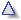Home DHTML-Drag&Drop-Library VectorGraphics-Library Tooltips-Library
 Online Function Grapher Written entirely in JavaScriptBy Walter Zorn
 Currently under development: A standalone application version of this Function Graphing Program, written in C language, much faster, essentially more capabilities, e.g. scrolling and zooming the displayed range, built-in function calculator, numerical integration, solving differential equations numerically ... free download of WZGrapher.
don't delete previous graphs
x min  x max          y min  y max
Function f(x) (up to 5 functions may be entered simultaneously, separated from each other by a semicolon)

Function Graph Area.

Operators and Helper Functions
upper/lower case is arbitrary
+ - * : /
() [] {}
Plus, minus, multiplication, division, grouping symbols. Multiplication sign * is not necessary and can be omitted. Examples: 0.5x^3-3x or pixeln(2cos[LN2x]) or Pipi/(Exe) Using different types of grouping symbols (parentheses, brackets, braces) for clarity of nested expressions is optional, but not necessary.
base^exponent
or
p(base,exponent)
Exponentiation baseexponent, for example p(x,2) or x^2 . Instead of ^ you can also type a single quote ' or a double quote ". If you don't use p(), make sure that bases or exponents composed by sub-expressions such as additions, subtractions, multiplications or divisions, are clearly marked off by being completely enclosed into parentheses, brackets or braces. Example: (x/3)^(2x) . Instead of x"3, of course, you can also enter xxx. Or, instead of tan(x)'2 you may type tan(x)tan(x).
root(arg,index) "index-th" root of argument. Example: root(x,6) sixth root of x, root[tan(x),4] fourth root of the tangent of x.
sqrt() Square root of argument (number or expression inside the parentheses). Equivalent to root(argument,2)
cbrt() Cube root of argument. Equivalent to root(argument,3)
logn(arg,base) Logarithm base-base of arg.
ln() Natural logarithm of argument (base-E logarithm of argument where E is Euler's constant)
lg() Logarithm base-10 of argument, equivalent to logn(argument,10).
lb() Logarithm base-2 of argument.
exp() Exponential Function E to the power of argument, equivalent to E^argument
sin() Sine of argument
cos() Cosine
tan() Tangent
cot() Cotangent
sec() Secant of argument, equiv. to 1/cos(arg).
csc() Cosecant, equiv. to 1/sin(arg).
asin() Arc sine
acos() Arc cosine
atan() Arc tangent
acot() Arc cotangent
asec() Arc secant, inverse secant.
acsc() Arc cosecant, inverse cosecant.
sinh() Hyperbolic sine, Sinus hyperbolicus
cosh() Hyperbolic cosine, Cosinus hyperbolicus
tanh() Hyperbolic tangent, Tangens hyperbolicus
coth() Hyperbolic cotangent, Cotangens hyperbolicus
sech() Hyperbolic secant, Secans hyperbolicus.
csch() Hyperbolic cosecant, Cosecans hyperbolicus.
asinh() Area sine, Area sinus hyperbolicus, inverse sinh().
acosh() Area cosine, Area cosinus hyperbolicus, inverse cosh().
atanh() Area tangent, Area tangens hyperbolicus, inverse tanh().
acoth() Area cotangent, Area cotangens hyperbolicus, inverse cotanh().
asech() Area- secant, Area secans hyperbolicus, inverse sech().
acsch() Area- cosecant, Area cosecans hyperbolicus, inverse csch().
gaussd(x,mean,sigma) Gaussian distribution ("Bell Curve"). gaussd(x,0,1), by the way, is the special case "Normal distribution density with mean-value=0, standard-deviation=1".
min(arg1,arg2) Returns the lesser of the two arguments
max(arg1,arg2) Returns the greater of the two arguments
round() Rounds argument up or down to the closest integer
floor() Rounds arg down.
ceil() Rounds arg up.
abs() or | | Absolute value of argument. Example: 2abs(sin[x]) or alternatively 2|sin(x)| .
sgn() Sign Function.
 sgn(x)  = 1 for x > 0 0 for x = 0 -1 for x < 0

Predefined Constants, Values
upper/lower case is arbitrary
 rand Random number between 0 und 1. Example:pi*rand*sin(x) or even Pirandsin(x) . E Euler's constant 2.718281828459045... LN2 Natural logarithm of 2, is 0.6931471805599453... LN10 Natural logarithm of 10, is 2.302585092994046... LOG2E Base-2 logarithm of E (E: see above), is 1.4426950408889633... LOG10E Base-10 logarithmus of E, is 0.4342944819032518... PHI Golden Ratio 1.61803398874989... PI 3.141592653589793... SQRT1_2 Square root of 1/2, is 0.7071067811865476... SQRT2 Square root of 2, is 1.4142135623730951...

Notes

Enter functions in standard mathematical notation, using x as independent variable. As evident from the examples on this page, arguments to helper functions must be enclosed into parentheses (or braces or brackets).

Grouping symbols such as parentheses, and helper functions may be arbitrarily deeply nested. Examples:
cos[atan(5x)^4] or sqrt{16*[1-sec(x)^2/4]}

The function plotter engine follows the common rules of math. notation and order of operation; for example, expressions inside parentheses have increased precedence, * and / have higher precedence than + and -, etc.. In case no grouping symbols are used, 2*x-3/x evaluates as if it were (2*x) - (3/x).

Since horizontal fraction lines aren't available to clearly mark off fraction expressions composed by sub-expressions, is is recommended to insert numerator and denominator each completely into parentheses (or brackets or braces). For example, type in pi-(5-x)/(3x)*acoth(x) instead of pi-5-x/3x*acoth(x) which otherwise would evaluate to pi-5-(x/3)*x*acoth(x).

Upper and lower case may be arbitrarily used and mixed, even for predefined constants. Spaces may be used for clarity as you wish, but neither help nor harm; the program engine ignores them.

Range of x (x min to x max) should not extend beyond minus 1012 and plus 1012. Range of y (y min to y max) is optional; if not specified, the function-grapher engine tries to evaluate it for the specified x range.

Multiple Functions at Once

You may enter up to 5 functions simultaneously, each terminated by a semicolon. Of course building the diagram then will take more time, possibly several seconds. Example (you may copy it into the function input):
sqrt[16*(1-x²/2)];
-sqrt[16*(1-x²/2)];
sqrt[2*(1-x²/16)];
-sqrt[2*(1-x²/16)]

Create Fourier Series

 The Fourier Series will be written into the lightblue area below. You may copy it from there, and enter it into the input of the Function Grapher   n: from to step width Single terms: Add subtract or alternate, beginning with plus minus Single term:Home